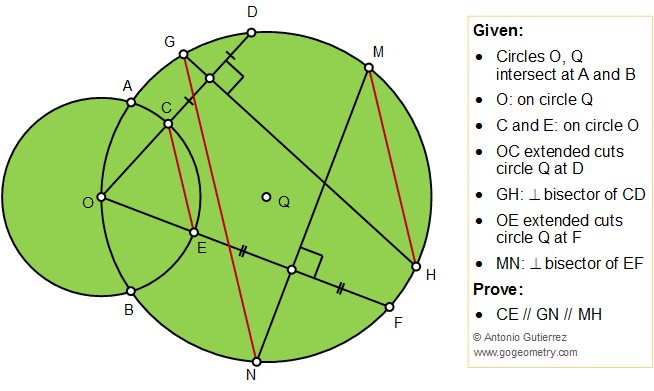## Friday, July 5, 2019

### Geometry Problem 1441 Intersecting Circles, Perpendicular Bisector, Parallel Lines

Geometry Problem. Post your solution in the comment box below.
Level: Mathematics Education, High School, Honors Geometry, College.

Details: Click on the figure below.1.https://photos.app.goo.gl/VcpDVKKDqwxshkUg9

Define points I, J, K, L, P, S,T, X and Y as per attached sketch
Note that I, J are midpoints of OD and CD
L and K are midpoints of OF and EF
QXJI and QYKL are rectangles
We have OJ= ½(OC+OD)= 1/2OC + OI
And JI= OJ-OI= 1/2OC= QX
Similarly, KL= ½ OE=1/2OC= QY
Since QX=QY => Chord GH= Chord MN=> Arc GH= Arc MN
And Arc GM=Arc NH => GN//MH
Let OS ⊥GN , we have quadrilaterals OSGJ and OSNK are cyclic
We have ∠ (COS)= ∠ (SGJ)= u=∠ (SNK)= ∠ (SOP)
So ∠ (COS)=u=∠ (SOE) => OS is the angle bisector of ∠ (TOP) and ∠ (COE)=> CE//GN

2.From previous problem, A,C,H co line, M,E,B co line.
Connect ACH, connect BEM.
Connect AB;
so angle AHM = angle ABM;
also angle CEM = angle ABE (ACEM on the same circle O),
so CE//MH

Let GN and OF cross at P;
OA=OB, so curve OA=curve OB;
curve AG= curve GD; curve BN=curve NF; so angle(OA)+angle(OB)+angel(AG)+angle(GD)+angle(DMHF)+angle(NF)+angle(BN)=180;
so angle NPF = angle(NF)+angle(OA)+angle(AG) = 90-1/2 angle(DMHF) =90- 1/2angleFOD = angle CEO.
So CE//GN;
So CE//GN//MH

3.If Point's
Q(R,0) and O(0,0)

Define:
(x-r)²+y²=R²
x²+y²=r²

On Point O, can define two lines
y=a*x (Line on Point's OCD) and y=b*x (Line on Point's OEF)

So:
(x-R)²+y²=R²
y=a*x
=> Point D [R/(1+a²),(a*R/(1+a²))]

(x-R)²+y²=R²
y=b*x
=> Point F [R/(1+b²),(b*R/(1+b²))]

x²+y²=R²
y=a*x
=> Point C [r²/(1+a²),(a*r²/(1+a²))]

x²+y²=R²
y=b*x
=> Point B [r²/(1+b²),(b*r²/(1+b²))]

Solve for the Slope k (germ. Steigung) at the Line on the Point's CE
k(1)=a-b (i)

On Point's CD can construction a Normal line, who will Intersect the (Big) Circle (-R,0)

n: y=-(1/a)*(x-(R+r²)/(2*(1+a²)))+a*(R+r²)/(2(1+a²))

=> The Intersect Point's:
G [(p+q)/(2(1+a²)),(a*(r²-R)-p/a)/(2(1+a²))] and H [(q-p)/(2(1+a²)),(a*(r²-R)+p/a)/(2(1+a²))]

The same procedure can be define the Point's M and N.
...
=> The Intersect Point's:
M [(u+v)/(2(1+b²)),(b*(r²-R)-u/b)/(2(1+b²))] and N [(v-u)/(2(1+b²)),(b*(r²-R)+u/b)/(2(1+b²))]

In wich:
p=a*√(R²(4a²+3)+r²(2R-r²)) , q=2a²R+R+r²

u=b*√(R²(4b²+3)+r²(2R-r²)) , v=2b²R+R+r²

__________

Now it can define a new Lines with Point's G and N, M and H.
The Slope from the new Lines, a solve will be show:

k(2)=a-b, line with Point's G and N (ii)
k(3)=a-b, line with Point's M and H (iii)
__________________________

So:
For the three Lines, the Slope is a same.
A prove for the Parallelity lines.

4.We use the results of problem n°1440 applied to both lines (O,C,D) and (O,E,F), and consider alignements (A,C,H) and (B,E,M) to which we apply Reim’s theorem (see problem n°72).
Thus: MH//CE.
Similarly we obtain alignements (A,E,N) and (B,C,G) resulting in GN//CE.